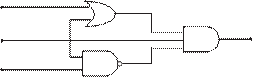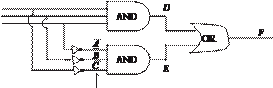### Create an Account

Home / Questions / For the logic circuit in Figure P12 35 write the logical expression for the outputs of thi...

# For the logic circuit in Figure P12 35 write the logical expression for the outputs of this circuit in terms of its inputs and create a truth table for the outputs in terms of the inputs including

For the logic circuit in Figure P12.35, write the logical expression for the outputs of this circuit in terms of its inputs, and create a truth table for the outputs in terms of the inputs, including any required intermediate variables.

Figure P12.31

12.32 A “consensus indicator” logic circuit is shown in Figure P12.32. Write the logical expression for the output of this circuit in terms of its input. Also create a truth table for the output in terms of the inputs.A

B C

Figure P12.35

OR

NAND

AND              FA B C

Jun 18 2020 View more View LessSubscribe To Get Solution